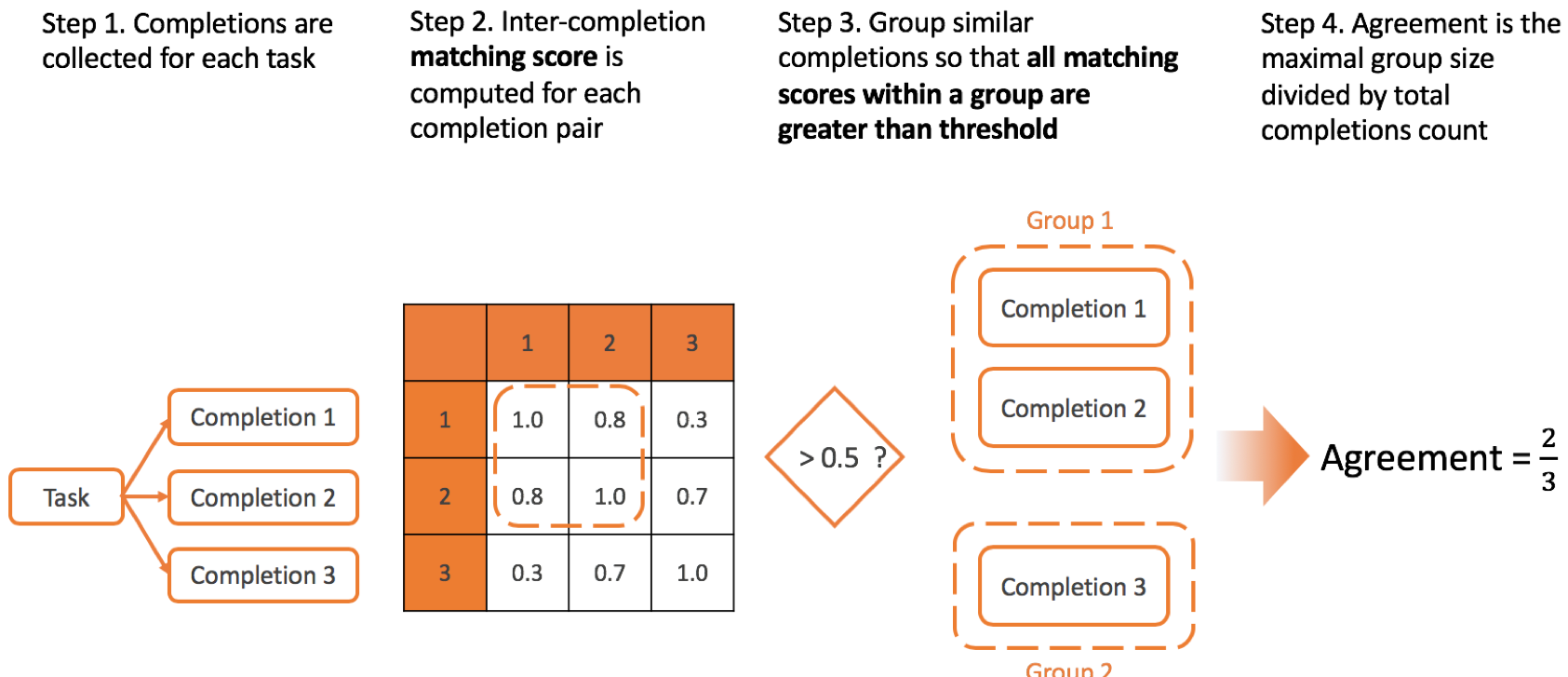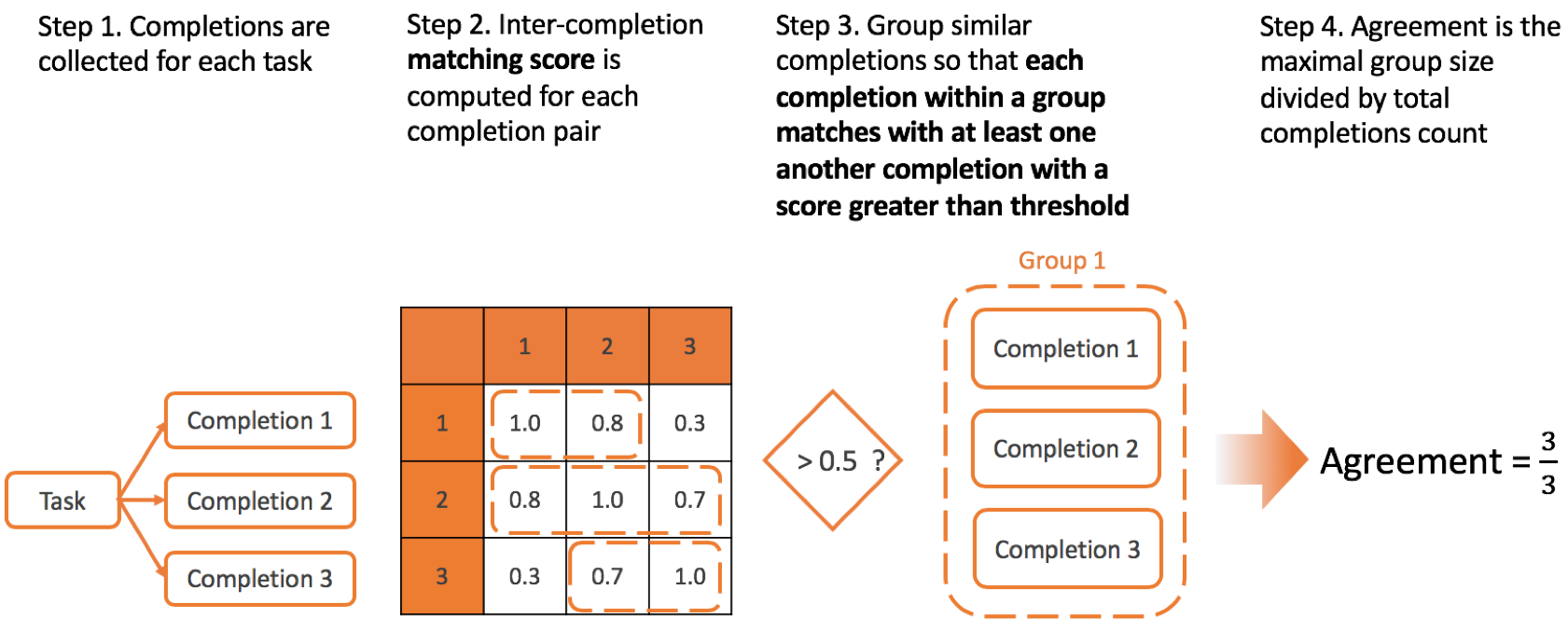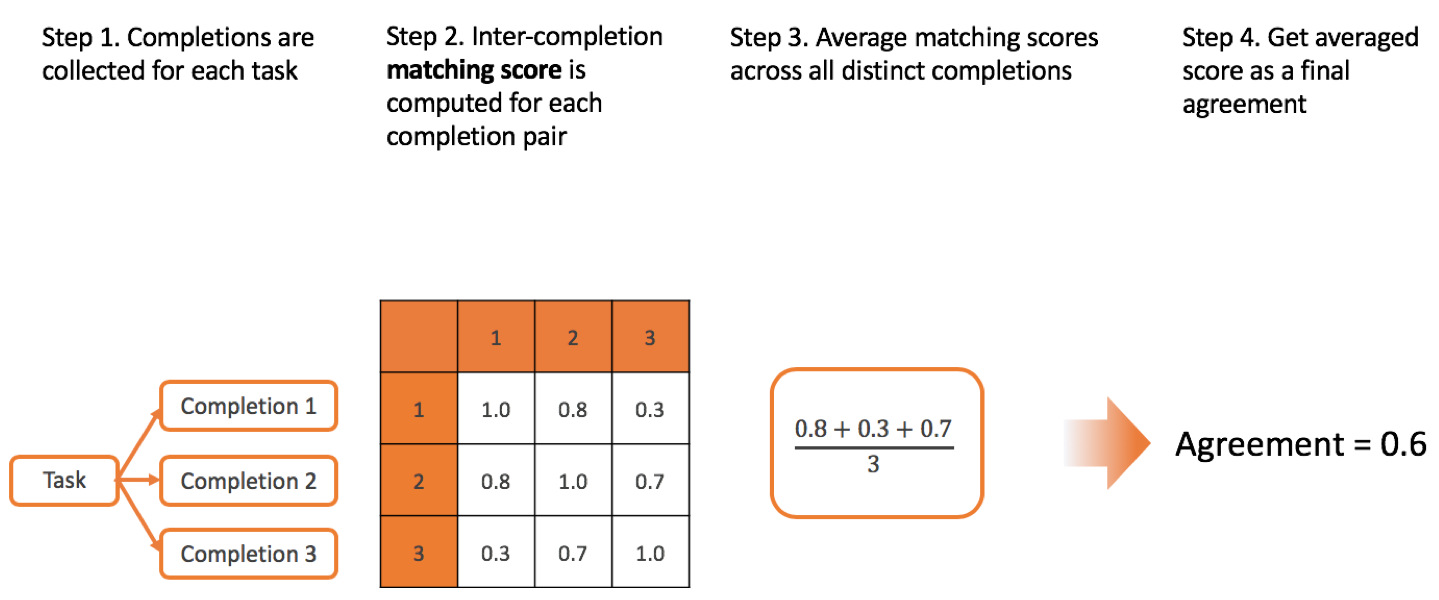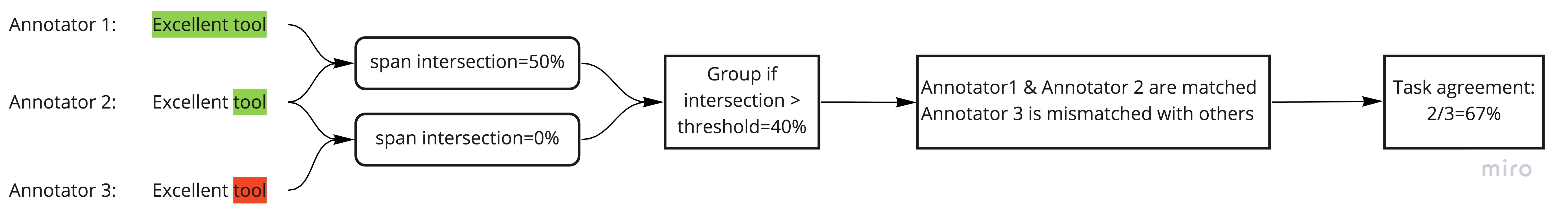# Statistics

Task agreement shows how multiple collaborators are in labeling consensus performing the same task. There are

• per-task agreement score on Data Manager page
• inter-annotator agreement matrix on `/business/projects/<project_id>/experts/` page

## Matching score

Each agreement matching score `m(x, y)` is computed differently depending on data labeling types presented in `x` and `y`,

• if `x` and `y` are both empty, `m(x, y) = 1`
• when no results with matching type found in `x` or `y`, `m(x, y) = 0`
• if there are different labeling types presented in `x` and/or `y`, partial matching scores for each data labeling type are averaged.
• for categorical labelings (e.g. `Choices` alone) Cohen’s Kappa index is computed if specified in project settings)

Currently we are using the following matching scores:

#### Choices

`m(x, y) = 1` if `x` and `y` are exact matched choices, and `m(x, y) = 0` otherwise

#### TextArea

Each TextArea result `x` or `y` contains list of texts, e.g. `x = [x1, x2, ..., xN]`. The matching score is computed by the following:

• For each aligned pair `(xi, yi)` the text edit normalized similarity is calculated.
• For unaligned pair (i.e. when one list of texts is longer than another), normalized similarity is zero.
• Similarity scores are averaged across all pairs.

The are several options available for this matching function:

Algorithm - edit distance algorithm

Split by - whether splits are taken by words or by chars

#### Labels

Intersection over resulted spans `m(x, y) = spans(x) ∩ spans(y)` normalized by total spans length

#### Rating

`m(x, y) = 1` if `x` and `y` are exact matched choices, and `m(x, y) = 0` otherwise

#### Ranker

Mean average precision (mAP)

#### RectangleLabels

There are several possible choices available on project settings page:

• Intersection over Union (IoU), averaged over all bounding boxes pairs with best match
• Precision computed for some threshold imposed on IoU
• Recall computed for some threshold imposed on IoU
• F-score computed for some threshold imposed on IoU

#### PolygonLabels

There are several possible choices available on project settings page:

• Intersection over Union (IoU), averaged over all polygon pairs with best match
• Precision computed for some threshold imposed on IoU
• Recall computed for some threshold imposed on IoU
• F-score computed for some threshold imposed on IoU

## Agreement method

Agreement method defines the way how matching scores between the all completions for one tasks are combined to form a single inter-annotator agreement score.

There are several possible methods you can specify on project settings page: